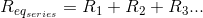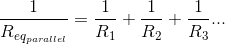# Resistor Network

## Working principles

• Voltage across a resistor generates a current.
• Current flows through the path of the least resistance.
• Resistors can be combined in series and parallel configurations.

### Applicable formulasFor two resistors in parallel with different values:If two resistances or impedances in parallel are of the same value, their equivalent resistance is half the value of one resistor, or R/2. For three equivalent resistors in parallel: R/3, and so on.

### Experiment

• What are the equivalent impedances for both branches?